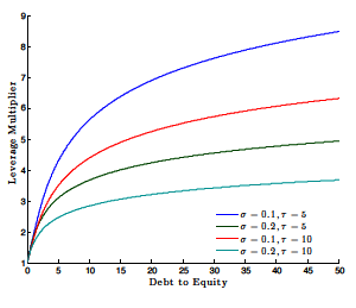# Structural GARCH: The Volatility-Leverage ConnectionThis paper proposes a new model of volatility featuring a “leverage multiplier” by which financial leverage amplifies equity volatility. The model estimates daily asset returns and asset volatility. (Working Paper no. 14-07)

#### Abstract

We propose a new model of volatility where financial leverage amplifies equity volatility by what we call the “leverage multiplier.” The exact specification is motivated by standard structural models of credit; however, our parametrization departs from the classic Merton (1974) model and can accommodate environments where the firm’s asset volatility is stochastic, asset returns can jump, and asset shocks are nonnormal. In addition, our specification nests both a standard GARCH and the Merton model, which allows for a statistical test of how leverage interacts with equity volatility. Empirically, the Structural GARCH model outperforms a standard asymmetric GARCH model for approximately 74 percent of the financial firms we analyze. We then apply the Structural GARCH model to two empirical applications: the leverage effect and systemic risk measurement. As a part of our systemic risk analysis, we define a new measure called “precautionary capital” that uses our model to quantify the advantages of regulation aimed at reducing financial firm leverage.